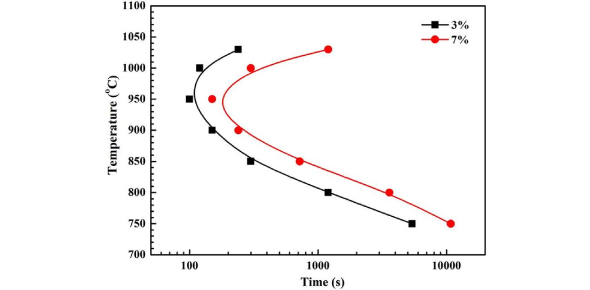# Ttt (Time Temperature Transformation) Diagram Quiz!

10 Questions | Total Attempts: 1033SettingsTime-Temperature-Transformation diagram is a method in material science for understanding the transformation of alloy steel. Here this quiz is specially designed for science students of this field. Let's test your level of knowledge on such a topic.

• 1.
The first step in constructing a TTT diagram involves _________ the sample.
• A.

Annealing

• B.

Normalizing

• C.

Quenching

• D.

Austenitizing

• 2.
TTT diagram is also called as:
• A.

S curve

• B.

C curve

• C.

Isothermal transformation curve

• D.

Baine's Diagram

• 3.
Non-equilibrium phases are shown for their time and transformation using
• A.

Fe- Fe3C diagram

• B.

TTT diagram

• C.

CCT diagram

• D.

TTT and CCT diagram

• 4.
At normal cooling rate ___________is formed.
• A.

Pearlite

• B.

Bainite

• C.

Martensite

• D.

Cementite

• 5.
______________is a definite carbide of iron which is extremely hard, being harder than ordinary hardened steel
• A.

Martensite

• B.

Cementite

• C.

Austenite

• D.

Ferrite

• 6.
The curve which gives the start time of transformation in TTT curve is called:
• A.

S curve

• B.

Phase diagram

• C.

C curve

• D.

CCT curve

• 7.
Lower critical temperature (A1) in iron carbon diagram is:
• A.

• B.

• C.

• D.

None

• 8.
Hardness of steel increases with the:
• A.

Increase of C %

• B.

Decrease of C %

• C.

Slow Cooling

• D.

None

• 9.
% of carbon in high carbon steel is:
• A.

0.5% to 1.0 %and

• B.

0.5 % t0 0.9%

• C.

0.5 % to 0.7 %

• D.

None

• 10.
On a two component solid-liquid phase diagram, a tie line indicates which of the following?
• A.

A region where the temperature is constant

• B.

A region where the composition is constant

• C.

An area below which only the solid phase exists

• D.

An area above which only the liquid phase exists

Related TopicsBack to top